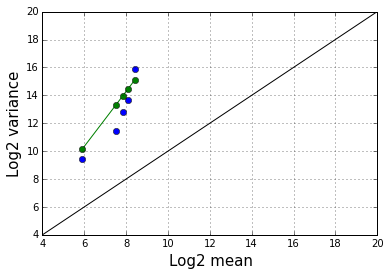# Fish abundance data¶

This notebook demonstrates Poisson and negative binomial regression in Statsmodels. We use a data set containing records of the number of fish caught at various sites in the ocean. At each site, we have the depth at which the fish were caught, and the density of foliage at the site. A description of the data is here, and the data are available here.

Here are the import statements that we need:

In :
%matplotlib inline

import pandas as pd
import numpy as np
import statsmodels.api as sm
import matplotlib.pyplot as plt


Next we read in the data set and check the number of rows and columns.

In :
data = pd.read_csv("fishing.csv", index_col=0)
print(data.shape)

(147, 7)


These are the variable names:

In :
data.dtypes

Out:
site           int64
totabund       int64
density      float64
meandepth      int64
year           int64
period        object
sweptarea    float64
dtype: object

Check for missing data.

In :
print(pd.isnull(data).sum())

site         0
totabund     0
density      0
meandepth    0
year         0
period       0
sweptarea    0
dtype: int64


The dependent variable for the regression analysis is totabund (the number of fish caught in a particular area). Count data are often right skewed, we next check the marginal distribution of the totabund values.

In :
data.totabund.plot(kind='hist')
plt.xlabel("Number of fish caught", size=15)
plt.ylabel("Frequency", size=15)

Out:
<matplotlib.text.Text at 0x7ffafdc7ea90>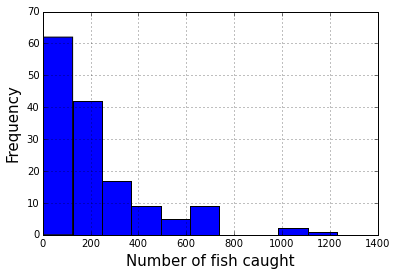Further complicating things, the regions where the fish are caught have different sizes. To make the totabund values comparable to each other, we can look at the number of fish caught per unit volume, as in the histogram below. However these values are not ideal for a regression model, because their variances differ based on the volumes, as well as on whatever intrinsic variation there is in the numbers of fish that are caught.

In :
(data.totabund / data.sweptarea).plot(kind='hist')
plt.xlabel("Number of fish caught per unit volume", size=15)
plt.ylabel("Frequency", size=15)

Out:
<matplotlib.text.Text at 0x7ffafa3bf438>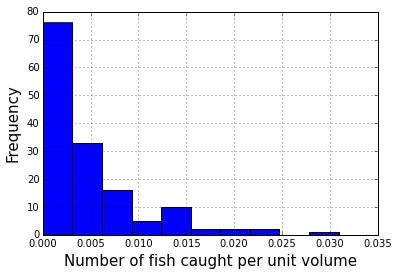Poisson regression

A better way to handle the unequal areas is to use a regression model with an "exposure" variable. The fitted values from the regression model based on the covariates are multiplied by the exposure value. The regresson model we will use is a Poisson GLM. In a Poisson GLM, the distribution of values at a given covariate vector has a Poisson distribution.

In :
model1 = sm.GLM.from_formula("totabund ~ meandepth + density", family=sm.families.Poisson(), exposure=data.sweptarea, data=data)
result1 = model1.fit()
print(result1.summary())

                 Generalized Linear Model Regression Results
==============================================================================
Dep. Variable:               totabund   No. Observations:                  147
Model:                            GLM   Df Residuals:                      144
Model Family:                 Poisson   Df Model:                            2
Link Function:                    log   Scale:                             1.0
Method:                          IRLS   Log-Likelihood:                -3174.8
Date:                Fri, 12 Jun 2015   Deviance:                       5377.1
Time:                        03:45:02   Pearson chi2:                 4.84e+03
No. Iterations:                     8
==============================================================================
coef    std err          z      P>|z|      [95.0% Conf. Int.]
------------------------------------------------------------------------------
Intercept     -4.9252      0.018   -267.167      0.000        -4.961    -4.889
meandepth     -0.0006   7.44e-06    -80.051      0.000        -0.001    -0.001
density       88.0607      0.739    119.087      0.000        86.611    89.510
==============================================================================


By default, the Poisson GLM uses an "exponential link function", meaning that the mean value is the exponential of the linear predictor. In the model above, the linear predictor is -4.9252 - 0.0006 * meandepth + 88.0607 * density. A consequence of using the exponential link function is that the covariate effects are multiplicative on the mean. If meandepth increases by 1 unit, the linear predictor changes additively by -0.0006 units, and the mean value changes by a factor of exp(-0.006).

An alternative to using sweptarea as the exposure is to include log(sweptarea) as a covariate. Using sweptarea as an exposure variable is exactly equivalent to using log(sweptarea) as a covariate and forcing its coefficient to be equal 1. It often happens that when allowing the coefficient of log(sweptarea) to be estimated, we discover that the fitted coefficient differs from 1, typically being smaller than 1. This means that the natural scaling is somewhat dampened -- if we double the search area, the yield does not quite double. There are various reasons why this would happen in the real world.

In :
model2 = sm.GLM.from_formula("totabund ~ meandepth + density + I(np.log(sweptarea))", family=sm.families.Poisson(), data=data)
result2 = model2.fit()
print(result2.summary())

                 Generalized Linear Model Regression Results
==============================================================================
Dep. Variable:               totabund   No. Observations:                  147
Model:                            GLM   Df Residuals:                      143
Model Family:                 Poisson   Df Model:                            3
Link Function:                    log   Scale:                             1.0
Method:                          IRLS   Log-Likelihood:                -3072.8
Date:                Fri, 12 Jun 2015   Deviance:                       5173.1
Time:                        03:45:02   Pearson chi2:                 4.63e+03
No. Iterations:                     8
========================================================================================
coef    std err          z      P>|z|      [95.0% Conf. Int.]
----------------------------------------------------------------------------------------
Intercept               -1.8025      0.218     -8.263      0.000        -2.230    -1.375
meandepth               -0.0005   9.32e-06    -55.097      0.000        -0.001    -0.000
density                 85.4746      0.764    111.933      0.000        83.978    86.971
I(np.log(sweptarea))     0.7004      0.021     33.550      0.000         0.660     0.741
========================================================================================


Overdispersion

An important property of the Poisson distribution is that the mean is equal to the variance. We can check this condition in our data by binning the data into quintiles based on the estimated means, and comparing the empirical mean and variance within each bin, as below.

In :
ii = np.digitize(result1.fittedvalues, np.percentile(result1.fittedvalues, [20, 40, 60, 80]))

moments = []
for i in range(5):
jj = np.flatnonzero(ii == i)
moments.append([result1.fittedvalues[jj].mean(), model1.endog[jj].var()])
moments = np.asarray(moments)

moments_log2 = np.log(moments) / np.log(2)

plt.grid(True)
plt.plot(moments_log2[:, 0], moments_log2[:, 1], 'o')
plt.plot([4, 20], [4, 20], '-', color='black')
plt.xlim(4, 20)
plt.ylim(4, 20)
plt.xlabel("Log2 mean", size=15)
plt.ylabel("Log2 variance", size=15)

Out:
<matplotlib.text.Text at 0x7ffafa311518>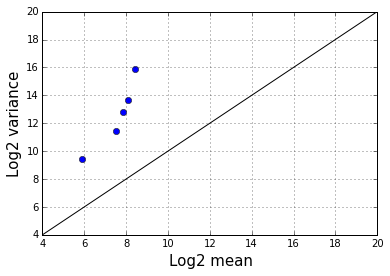The plot above indicates that the variance is at least four times greater than the mean, so the key condition of the Poisson distribution does not hold. Another way to check this is to estimate the "scale parameter", which should be equal to 1 for a Poisson model. In this case, however, it is estimated to be 33. When data have conditional variance much larger than the conditional mean in a Poisson model, this is called "overdispersion".

In :
model3 = sm.GLM.from_formula("totabund ~ meandepth + density", family=sm.families.Poisson(), exposure=data.sweptarea, data=data)
result3 = model3.fit(scale='x2')
print(result3.summary())

                 Generalized Linear Model Regression Results
==============================================================================
Dep. Variable:               totabund   No. Observations:                  147
Model:                            GLM   Df Residuals:                      144
Model Family:                 Poisson   Df Model:                            2
Link Function:                    log   Scale:                   33.6142543859
Method:                          IRLS   Log-Likelihood:            -1.0672e+05
Date:                Fri, 12 Jun 2015   Deviance:                       5377.1
Time:                        03:45:03   Pearson chi2:                 4.84e+03
No. Iterations:                     8
==============================================================================
coef    std err          z      P>|z|      [95.0% Conf. Int.]
------------------------------------------------------------------------------
Intercept     -4.9252      0.107    -46.081      0.000        -5.135    -4.716
meandepth     -0.0006   4.31e-05    -13.807      0.000        -0.001    -0.001
density       88.0607      4.287     20.540      0.000        79.658    96.464
==============================================================================


If we fix the scale parameter at 1 (which is the default value for the Poisson family), we have much smaller standard errors, but these are misleading (note that the parameter estimates are the same, only the standard errors change).

In :
model4 = sm.GLM.from_formula("totabund ~ meandepth + density", family=sm.families.Poisson(), exposure=data.sweptarea, data=data)
result4 = model4.fit()
print(result4.summary())

                 Generalized Linear Model Regression Results
==============================================================================
Dep. Variable:               totabund   No. Observations:                  147
Model:                            GLM   Df Residuals:                      144
Model Family:                 Poisson   Df Model:                            2
Link Function:                    log   Scale:                             1.0
Method:                          IRLS   Log-Likelihood:                -3174.8
Date:                Fri, 12 Jun 2015   Deviance:                       5377.1
Time:                        03:45:03   Pearson chi2:                 4.84e+03
No. Iterations:                     8
==============================================================================
coef    std err          z      P>|z|      [95.0% Conf. Int.]
------------------------------------------------------------------------------
Intercept     -4.9252      0.018   -267.167      0.000        -4.961    -4.889
meandepth     -0.0006   7.44e-06    -80.051      0.000        -0.001    -0.001
density       88.0607      0.739    119.087      0.000        86.611    89.510
==============================================================================


To further clarify the concept of overdispersion, we simulate perfect Poisson data and estimate the scale parameter. It is reasonably close to 1 (if the sample size is not large, the estimate will vary a lot, but the mean value is still very close to 1). Note also that the regression coefficient of x is accurately estimated.

In :
x = np.random.normal(size=1000)
mn = np.exp(0.2 * x)
y = np.random.poisson(mn)

model4 = sm.GLM(y, x, family=sm.families.Poisson())
result4 = model4.fit(scale='x2')
print(result4.summary())

                 Generalized Linear Model Regression Results
==============================================================================
Dep. Variable:                      y   No. Observations:                 1000
Model:                            GLM   Df Residuals:                      999
Model Family:                 Poisson   Df Model:                            0
Link Function:                    log   Scale:                  0.985665138387
Method:                          IRLS   Log-Likelihood:                -1277.9
Date:                Fri, 12 Jun 2015   Deviance:                       1109.2
Time:                        03:45:03   Pearson chi2:                     985.
No. Iterations:                     7
==============================================================================
coef    std err          z      P>|z|      [95.0% Conf. Int.]
------------------------------------------------------------------------------
x1             0.1836      0.031      5.987      0.000         0.124     0.244
==============================================================================


Negative binomial regression

A negative binomial GLM is a GLM for non-negative dependent variables (like Poisson regression). Also like Poisson regression, it is most often used with an exponential mean structure. However unlike in Poisson regression, the mean is not equal to the variance. Instead, the variance has the form m + a*m^2, for a positive parameter a that we can choose or fit to the data. Below we use maximum likelihood to estimate a.

In :
loglike = []
a_values = np.linspace(0.1, 1.5, 20)
for a in a_values:
model = sm.GLM.from_formula("totabund ~ meandepth + density", family=sm.families.NegativeBinomial(alpha=a), exposure=data.sweptarea, data=data)
result = model.fit()
loglike.append(result.llf)

loglike = np.asarray(loglike)
plt.plot(a_values, loglike)
plt.ylabel("Log likelihood", size=15)
plt.xlabel("Negative binomial parameter", size=15)

Out:
<matplotlib.text.Text at 0x7ffafa2725f8>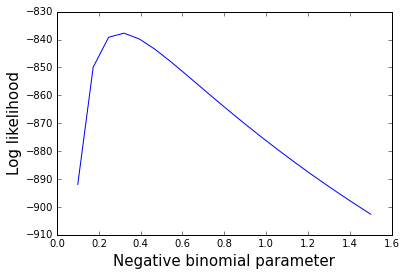The estimate of a is around 0.3, meaning that the variance is roughly equal to m + 0.3*m^2.

We can plot the fitted negative bnomial variance pattern together with the empirical means and variances in the quintiles of data. The agreement is quite good.

In :
plt.grid(True)
plt.plot(moments_log2[:, 0], moments_log2[:, 1], 'o')
va_log2 = np.log(moments[:, 0] + 0.3*moments[:, 0]**2)/np.log(2)
plt.plot(moments_log2[:, 0], va_log2, '-o')
plt.plot([4, 20], [4, 20], '-', color='black')
plt.xlim(4, 20)
plt.ylim(4, 20)
plt.xlabel("Log2 mean", size=15)
plt.ylabel("Log2 variance", size=15)

Out:
<matplotlib.text.Text at 0x7ffafa26bdd8>